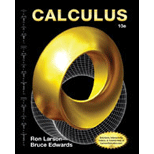# The volume V and the weight W of the beam where x = 2 1 + y 2 , x = − 2 1 + y 2 , y = 0 and y = 3### Calculus

10th Edition
Ron Larson + 1 other
Publisher: Cengage Learning
ISBN: 9781285057095### Calculus

10th Edition
Ron Larson + 1 other
Publisher: Cengage Learning
ISBN: 9781285057095

#### Solutions

Chapter 8.6, Problem 72E

(a)

To determine

## To calculate: The volume V and the weight W of the beam where x=21+y2,x=−21+y2,y=0 and y=3

(b)

To determine

### Want to see the full answer?

Check out a sample textbook solution.

### Want to see this answer and more?

Experts are waiting 24/7 to provide step-by-step solutions in as fast as 30 minutes!*

*Response times may vary by subject and question complexity. Median response time is 34 minutes for paid subscribers and may be longer for promotional offers.